# Upper Bound On A Determinant

In competitive prediction it is often necessary to find upper bounds for the determinant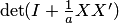, which, by the Sylvester identity, equals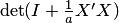. Let us assume that the size of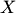is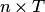and the columns ofare vectors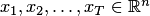; it is natural for the purposes of machine learning to formulate bounds involving properties of these vectors.

# Infinity Norm

A bound in terms of the infinity norms of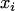was obtained in [Vovk]. Recall that the infinity norm of a vector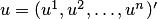is the maximum absolute value of its coordinates,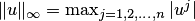.

The determinant of a positive definite matrix does not exceed the product of its diagonal elements (see, e.g., [Beckenbach and Bellman, Chapter 2, Theorem 7]. Let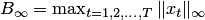; in other words,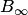is the maximum absolute value of an element of. A diagonal element of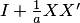does not exceed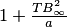and we get the bound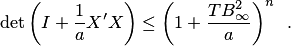In [Cesa-Bianchi et al] a bound in terms of the quadratic norms ofwas obtained. The quadratic (or Euclidean) norm of a vectoris given by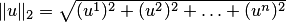.

The determinant of a matrix is the product of its (generally speaking complex) eigenvalues counting multiplicities. If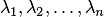are the eigenvalues of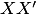, then the eigenvalues ofare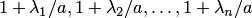and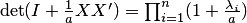.

The sum of eigenvalues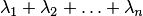equals the trace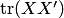and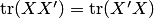. Indeed, the matrices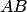and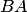(provided they exist) have the same non-zero eigenvalues counting multiplicities while zero eigenvalues do not contribute to the trace. Alternatively one can verify the equality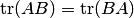by a straightforward calculation, see, e.g., [Axler, Proposition 10.9 (p. 219)]. The matrix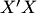is the Gram matrix of vectors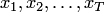and the elements on its diagonal are the squared quadratic norms of the vectors. Letting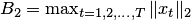we get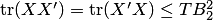.

The problem has reduced to obtaining an upper bound on the product of some positive numbers with a known sum. The inequality of arithmetic and geometric means implies that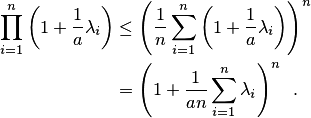Combining this with the bound on the trace yields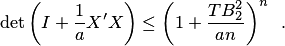# Comparison

Let us compare the bounds. For every vector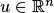we have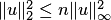(the inequality is tight: for a vector of equal coordinates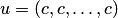it is an equality) and therefore for any array of vectorswe get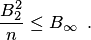We conclude that the quadratic norm bound is not weaker than the infinity norm bound.

# References

[Vovk] V. Vovk. Competitive on-line statistics. International Statistical Review, 69(2):213-248, 2001.

[Beckenbach and Bellman] E.F.Beckenbach and R.E.Bellman. Inequalities. Springer, 1961.

[Cesa-Bianchi et al] N.Cesa-Bianchi, A.Conconi, and C.Gentile. A second-order perceptron algorithm. SIAM Journal on Computing, 34(3):640-668, 2005.

[Axler] S.Axler. Linear Algebra Done Right. Springer, 2nd edition, 1997.